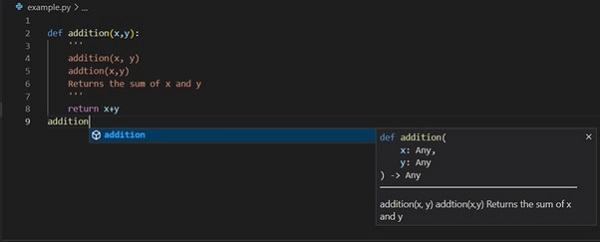# Python - Docstrings

In Python, a docstring is a string literal that serves as the documentation of different Python objects such as functions, modules, class as well as its methods and packages. It is the first line in the definition of all these constructs and becomes the value of __doc__ attribute.

### DocString of a Function

```def addition(x, y):
'''This function returns the sum of two numeric arguments'''
return x+y
```

It will produce the following output

```Docstring of addition function: This function returns the sum of two numeric arguments
```

The docstring can be written with single, double or triple quotation marks. However, most of the times you may want a descriptive text as the documentation, so using triple quotes is desirable.

All the built-in modules and functions have the __doc__ property that returns their docstring.

### Docstring of math module

```import math

print ("Docstring of math module:", math.__doc__)
```

It will produce the following output

```Docstring of math module: This module provides access to the mathematical functions
defined by the C standard.
```

### Docstring of Built-in functions

Following code displays the docstring of abs() function and randint() function in random module.

```print ("Docstring of built-in abs() function:", abs.__doc__)
import random

print ("Docstring of random.randint() function:",
random.randint.__doc__)
```

It will produce the following output −

```Docstring of built-in abs() function: Return the absolute value of the
argument.

Docstring of random.randint() function: Return random integer in range
[a, b], including both end points.
```

### Docstring of built-in class

Docstrings of built-in classes are usually more explanatory, hence the text is over multiple lines. Below, we check the docstring of built-in dict class

```print ("Docstring of built-in dict class:", dict.__doc__)
```

It will produce the following output

```Docstring of built-in dict class: dict() -> new empty dictionary
dict(mapping) -> new dictionary initialized from a mapping object's
(key, value) pairs
dict(iterable) -> new dictionary initialized as if via:
d = {}
for k, v in iterable:
d[k] = v
dict(**kwargs) -> new dictionary initialized with the name=value pairs in the keyword argument list. For example: dict(one=1, two=2)
```

### Docstring of Template class

Template class is defined in string module of Python's standard library. Its docstring is as follows −

```from string import Template

print ("Docstring of Template class:", Template.__doc__)
```

It will produce the following output

```Docstring of Template class: A string class for supporting \$- substitutions.
```

### Docstring in help system

The docstring is also used by Python's built-in help service. For example check its help of abs() function in Python interpreter −

```>>> help (abs)
Help on built-in function abs in module builtins:
abs(x, /)
Return the absolute value of the argument.
```

Similarly, define a function in the interpreter terminal and run help command.

```>>> def addition(x,y):
... Returns the sum of x and y
... '''
... return x+y
...
Help on function addition in module __main__:
Returns the sum of x and y
```

Docstring also is used by IDEs to provide useful type ahead information while editing the code.### Docstring as Comment

A string literal appearing anywhere other than these objects (function, method, class, module or package) is ignored by the interpreter, hence they are similar to comments (which start with # symbol).

```# This is a comment
print ("Hello World")
'''This is also a comment'''
print ("How are you?")
```